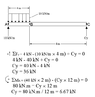# Statics Question -- Analyzing the forces on this supported beam

Thread moved from the technical forums to the schoolwork forums
Can someone please tell me why I get two different result of the value of Cy (please see attached image for more detail)?

Am I doing a mistake?

If yes, what is my mistake?

#### Attachments

•Statics.PNG
6.3 KB · Views: 98
In your analysis of Fy, where does the 4kN come from? It isn't defined in the figure. But if the analysis is to be correct then it is a force in the positive y direction (upward) at point A, so let's go with that.

Why would the two be equal? There is no law that says that this must be so. The thing you showed that if you want the Y-forces to be zero, then there is still a net moment, and if you want the moment to be zero, then there is still a net force.

In other words, there is no Cy to be found where the beam is in equilibrium.

•Lnewqban
It seems to be a simple beam with two vertical supports, one at each end.
If that is the case:
1) There is no reason for reaction at A to be in KN/m.
2) The magnitude of that reaction at A must be greater than the magnitude of the reaction at C, because the load is off center and loading A more than C.
3) The given values of reaction at A, 10 or 4, must be incorrect, since the distributed load can be considered as a puntual load of 40 KN located 2 meters from A.